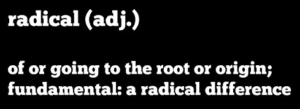## Simplifying Square Roots with Variables

### Learning Outcomes

• Simplify square roots with variables
• Recognize that by definition $\sqrt{x^{2}}$ is always nonnegative

Radical expressions are expressions that contain radicals. Radical expressions come in many forms, from simple and familiar, such as$\sqrt{16}$, to quite complicated, as in $\sqrt{250{{x}^{4}}y}$. Using factoring, you can simplify these radical expressions, too.## Simplifying Square Roots

Radical expressions will sometimes include variables as well as numbers. Consider the expression $\sqrt{9{{x}^{6}}}$. Simplifying a radical expression with variables is not as straightforward as the examples we have already shown with integers.

Consider the expression $\sqrt{{{x}^{2}}}$. This looks like it should be equal to x, right? Let’s test some values for x and see what happens.

In the chart below, look along each row and determine whether the value of x is the same as the value of $\sqrt{{{x}^{2}}}$. Where are they equal? Where are they not equal?

After doing that for each row, look again and determine whether the value of $\sqrt{{{x}^{2}}}$ is the same as the value of $\left|x\right|$.

$x$ $x^{2}$ $\sqrt{x^{2}}$ $\left|x\right|$
$−5$ $25$ $5$ $5$
$−2$ $4$ $2$ $2$
$0$ $0$ $0$ $0$
$6$ $36$ $6$ $6$
$10$ $100$ $10$ $10$

Notice—in cases where x is a negative number, $\sqrt{x^{2}}\neq{x}$! (This happens because the process of squaring the number loses the negative sign, since a negative times a negative is a positive.) However, in all cases $\sqrt{x^{2}}=\left|x\right|$. You need to consider this fact when simplifying radicals that contain variables, because by definition $\sqrt{x^{2}}$ is always nonnegative.

### Taking the Square Root of a Radical Expression

When finding the square root of an expression that contains variables raised to a power, consider that $\sqrt{x^{2}}=\left|x\right|$.

Examples: $\sqrt{9x^{2}}=3\left|x\right|$, and $\sqrt{16{{x}^{2}}{{y}^{2}}}=4\left|xy\right|$

Let’s try it.
The goal is to find factors under the radical that are perfect squares so that you can take their square root.

### Example

Simplify. $\sqrt{9{{x}^{6}}}$

Variable factors with even exponents can be written as squares. In the example above, ${{x}^{6}}={{x}^{3}}\cdot{{x}^{3}}={\left|x^3\right|}^{2}$ and

${{y}^{4}}={{y}^{2}}\cdot{{y}^{2}}={\left(|y^2\right|)}^{2}$.

### Try It

Let’s try to simplify another radical expression.

### Example

Simplify. $\sqrt{100{{x}^{2}}{{y}^{4}}}$

You can check your answer by squaring it to be sure it equals $100{{x}^{2}}{{y}^{4}}$.

### Example

Simplify. $\sqrt{49{{x}^{10}}{{y}^{8}}}$

You find that the square root of $49{{x}^{10}}{{y}^{8}}$ is $7\left|{{x}^{5}}\right|{{y}^{4}}$. In order to check this calculation, you could square $7\left|{{x}^{5}}\right|{{y}^{4}}$, hoping to arrive at $49{{x}^{10}}{{y}^{8}}$. And, in fact, you would get this expression if you evaluated ${\left({7\left|{{x}^{5}}\right|{{y}^{4}}}\right)^{2}}$.

In the video that follows we show several examples of simplifying radicals with variables.

### Example

Simplify. $\sqrt{{{a}^{3}}{{b}^{5}}{{c}^{2}}}$

In the next section, we will explore cube roots, and use the methods we have shown here to simplify them. Cube roots are unique from square roots in that it is possible to have a negative number under the root, such as $\sqrt{-125}$.

## Contribute!

Did you have an idea for improving this content? We’d love your input.